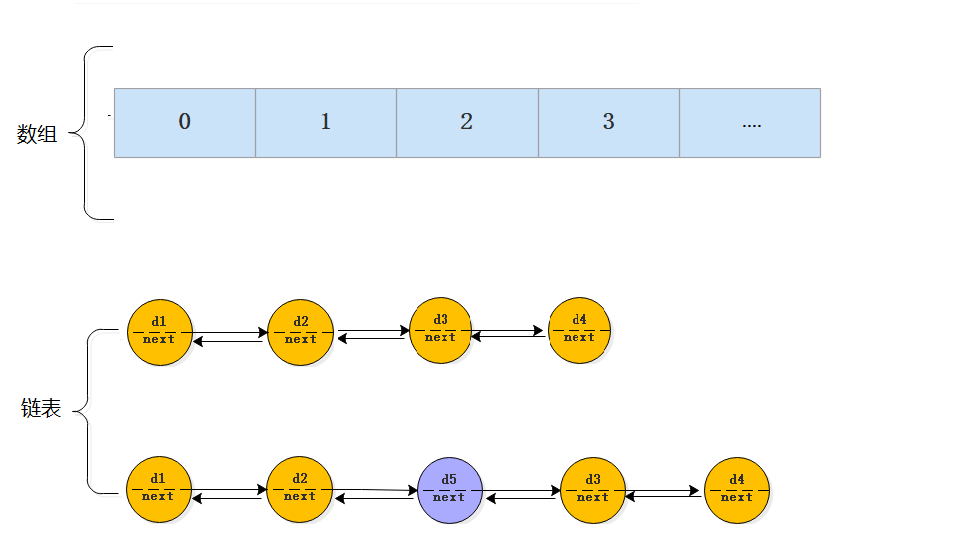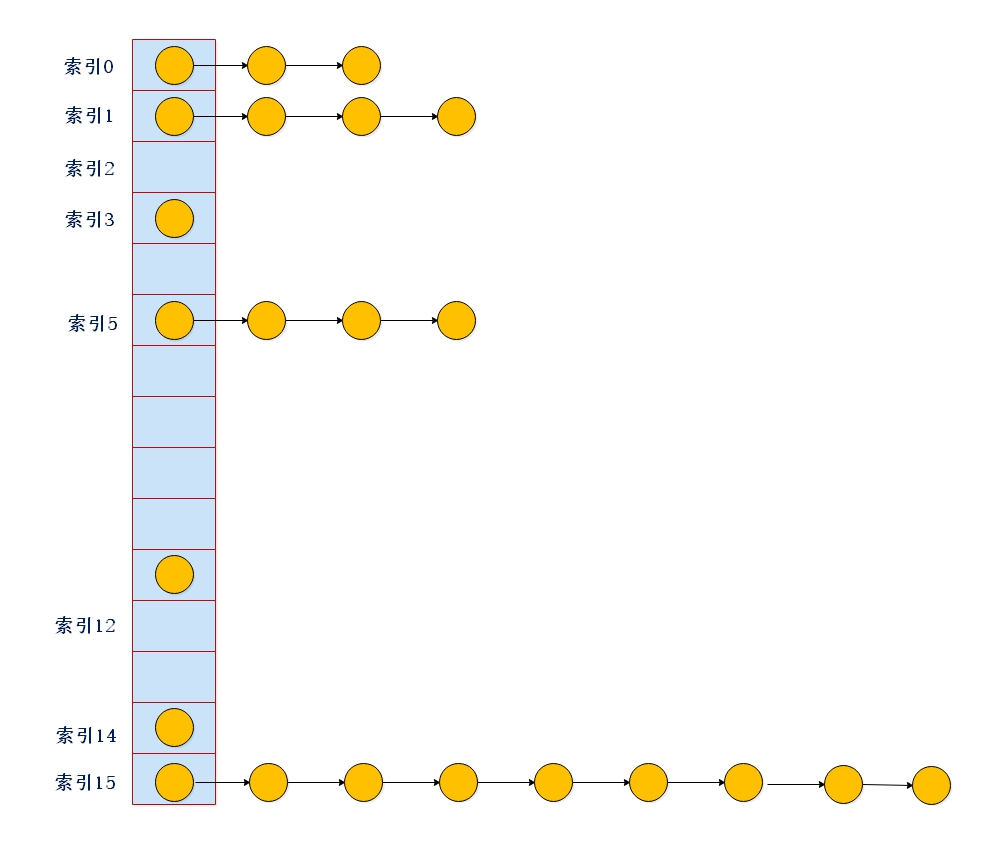# 不可不知的哈希映射

``````引言
hashmap这个东西呢，太老生常谈了

``````

## 2.1 HashMap数据结构

HashMap 概念、数据结构回顾（JDK8和JDK7） & 为什么1.8使用红黑树？

HashMap 是一个利用散列表（哈希表）原理来存储元素的集合，是根据Key value而直接进行访问的数据结构``````为什么是JDK1.8 是数组+链表+红黑树？？？
``````

HashMap变化历程

1.7的数据结构：链表变长，效率低 了！！！1.8的数据结构：JDK1.8使用红黑树，其实就是为了提高查询效率

## 2.2 HashMap继承体系HashMap已经继承了AbstractMap而AbstractMap类实现了Map接口

• Cloneable 空接口，表示可以克隆
• Serializable 序列化
• AbstractMap 提供Map实现接口

## 2.3 HashMap源码深度剖析

1）目标：

（1）HashMap的底层数据结构是什么？

（2）HashMap中增删改查操作的底部实现原理是什么？

（3）HashMap是如何实现扩容的？

（4）HashMap是如何解决hash冲突的？

（5）HashMap为什么是非线程安全的？

2）测试代码如下

``````package com.mmap;

import org.openjdk.jol.info.ClassLayout;

import java.util.ArrayList;
import java.util.ConcurrentModificationException;
import java.util.HashMap;
import java.util.List;
import java.util.concurrent.ConcurrentHashMap;

public class MMapTest {
public static void main(String[] args) {
HashMap<Integer, String> m = new HashMap<Integer, String>();//尾插
//断点跟踪put
m.put(1, "001");
m.put(1, "002");
m.put(17, "003");//使用17可hash冲突（存储位置相同）

System.out.println(ClassLayout.parseInstance(m).toPrintable());
//断点跟踪get
System.out.println(m.get(1));//返回002（数组查找）
System.out.println(m.get(17));//返回003（链表查找）
//断点跟踪remove
m.remove(1);//移除
System.out.println(m);
m.remove(1, "002");//和上面的remove走的同一个代码
}
}

``````

3）关于hashMap基本结构的验证### 2.3.1 成员变量与内部类

1<<4

``````
static final int DEFAULT_INITIAL_CAPACITY = 1 << 4; //16，默认数组容量：左位移4位，即16
static final int MAXIMUM_CAPACITY = 1 << 30;//最大容量：即2的30次幂
static final float DEFAULT_LOAD_FACTOR = 0.75f;//负载因子：扩容使用，统计学计算出的最合理的
static final int TREEIFY_THRESHOLD = 8;//链表转红黑树阈值
static final int UNTREEIFY_THRESHOLD = 6;//当链表的值小<6, 红黑树转链表

……

transient Node<K,V>[] table;//HashMap中的数组,中间状态数据
transient Set<Map.Entry<K,V>> entrySet;//用来存放缓存,中间状态数据;

transient int size;//size为HashMap中K-V的实时数量（重点）,注意！不是table的长度！
transient int modCount;//用来记录HashMap的修改次数，几个集合里都有它
``````

`````` static class Node<K,V> implements Map.Entry<K,V> {//数组和链表上的节点，1.8前叫Entry
final int hash;//扰动后的hash
final K key;//map的key
V value;//map的value
Node<K,V> next;//下个节点

Node(int hash, K key, V value, Node<K,V> next) { //构造函数，没啥说的
this.hash = hash;
this.key = key;
this.value = value;
this.next = next;//链表下一个
}
}

``````

### 2.3.2 HashMap构造器

1）目标：学习下面的三个构造器，它们都干了哪些事情？

``````    public HashMap() {
}
``````
``````    public HashMap(int initialCapacity) {
}
``````
``````    public HashMap(int initialCapacity, float loadFactor) {
//赋值，多了一些边界判断
if (initialCapacity < 0)
throw new IllegalArgumentException("Illegal initial capacity: " +
initialCapacity);
if (initialCapacity > MAXIMUM_CAPACITY)
initialCapacity = MAXIMUM_CAPACITY;
throw new IllegalArgumentException("Illegal load factor: " +
this.threshold = tableSizeFor(initialCapacity);  // 注意，map里是没有capacity这个类变量的！
}
``````

map的构造函数就做了几个赋值这么点事？这么简单？错！接着往下看

2）无参构造函数验证

put之前：3）自定义初始化参数验证``````		//capacity函数，初始化了table，就是table的length，否则取的是threshold
final int capacity() {
return (table != null) ? table.length :
(threshold > 0) ? threshold :
DEFAULT_INITIAL_CAPACITY;
}

//带参数的初始化，其实threshold调用的是以下函数：
//这是什么神操作？？？
//其实是将n转成2进制，右移再和自己取或，相当于把里面所有的0变成了1
//最终目的：找到>=n的，1开头后面全是0的数。如果n=111 ， 那就是 1000 ； 如果n=100，那就是它自己
//而这个数，恰好就是2的指数，为后面的扩容做铺垫
static final int tableSizeFor(int cap) {
int n = cap - 1;
n |= n >>> 1;
n |= n >>> 2;
n |= n >>> 4;
n |= n >>> 8;
n |= n >>> 16;  //到这一步n已经各个位都是1了。
//范围校验，小于0返回1，大于最大值返回最大值，绝大多数正常情况下，返回n+1，也就是10000……
return (n < 0) ? 1 : (n >= MAXIMUM_CAPACITY) ? MAXIMUM_CAPACITY : n + 1;
}

``````

``````package com.mmap;

public class TableSizeTest {

public static void main(String[] args) {
System.out.println(tableSizeFor(9));  //9的二进制更能看出以下变化
}

static final int tableSizeFor(int cap) {
System.out.println(Integer.toBinaryString(cap));  //1001
int n = cap - 1;
System.out.println(Integer.toBinaryString(n)); //1000
n |= n >>> 1;   //无符号右移，前面补0
System.out.println(Integer.toBinaryString(n)); //右移再或，1100
n |= n >>> 2;
System.out.println(Integer.toBinaryString(n)); //再移动2位， 1111
n |= n >>> 4;
System.out.println(Integer.toBinaryString(n)); //就这么长，再迁移也是1111
n |= n >>> 8;
System.out.println(Integer.toBinaryString(n));
n |= n >>> 16;
System.out.println(Integer.toBinaryString(n)); //Integer的最大长度32位，16折半后迁移全覆盖
System.out.println(Integer.toBinaryString(n+1));
return  n + 1;  //+1后变为 10000 ，也就是16 ， 2的4次方
}
}

``````

4）总结：

map的构造函数没有你想象的那么简单！

• 无参构造时，容量=16，因子=0.75。第一次插入数据时，才会初始化table、阈值等信息
• 有参构造时，不会容忍你胡来，会取大于但是最接近你容量的2的指数倍（想一下为什么？提示：和扩容规则有关）
• 无论哪种构造方式，扩容阈值最终都是 =（容量*因子）

### 2.3.3 HashMap插入方法

1）先了解下流程图2）关于key做hash值的计算

``````   public V put(K key, V value) {
return putVal(hash(key), key, value, false, true);//调用Map的putVal方法
}
``````

``````    static final int hash(Object key) {
int h;
//【知识点】hash扰动
return (key == null) ? 0 : (h = key.hashCode()) ^ (h >>> 16);
}

``````3）核心逻辑

``````    final V putVal(int hash, K key, V value, boolean onlyIfAbsent,
boolean evict) {//onlyIfAbsent：true不更改现有值；evict：false表示table为创建状态
//几个临时变量：
//tab=数组，p=插槽指针，n=tab的长度，i数组下标
Node<K,V>[] tab; Node<K,V> p; int n, i;
if ((tab = table) == null || (n = tab.length) == 0)//数组是否null或者==0，第1次put为空
//初始化数组(or扩容)，所以table是在这里初始化的，不是new的时候！
//初始时，n=16
n = (tab = resize()).length;  // resize，下面单独讲扩容

//【知识点】为何1 与 17 在一个槽上！秘密就藏在寻址这里
if ((p = tab[i = (n - 1) & hash]) == null)//寻址：(n - 1) & hash（重要！）
tab[i] = newNode(hash, key, value, null);//当前插槽没有值，空的！将新node直接扔进去
else {  //有值，说明插槽上发生了碰撞，需要追加成链表了！
//还是临时变量
//e=是否找到与当前key相同的节点，找到说明是更新，null说明是新key插入
//k=临时变量，查找过程中的key在这里暂存用
Node<K,V> e; K k;
if (p.hash == hash &&  //如果正好，插槽第一个节点（p），跟插入的key相同
((k = p.key) == key || (key != null && key.equals(k))))
e = p; //就将它赋值给e，注意！这时候还没覆盖上去，只是标记到e，发现了相同key的节点！
else if (p instanceof TreeNode) //如果不是这个key，但是类型是一个红黑树节点
//这说明当前插槽的链很长，已经变成红黑树了，就调putTreeVal，扔到这颗树上去
//树的插入，这里不赘述，在数据结构课中有
e = ((TreeNode<K,V>)p).putTreeVal(this, tab, hash, key, value);
else {//如果都不是以上情况，那就是链表了
for (int binCount = 0; ; ++binCount) {
if ((e = p.next) == null) {//顺着链表一直往后跳，直到遍历到尾巴节点
p.next = newNode(hash, key, value, null);//然后把key封装成新node追加到尾巴上
if (binCount >= TREEIFY_THRESHOLD - 1) //链表长度计数如果>8转红黑树
treeifyBin(tab, hash);//转成树
break;
}
if (e.hash == hash &&
((k = e.key) == key || (key != null && key.equals(k))))
break;// 如果遍历过程中找到相同key，那就赋给e，break跳出for循环，执行后面的逻辑
p = e;
}
}
if (e != null) { // 如果e非空，说明前面一顿猛如虎的操作后，找到了相同的key
V oldValue = e.value;
if (!onlyIfAbsent || oldValue == null)
e.value = value;// 看看onlyIfAbsent，如果让覆盖那就覆盖，不让那就算了
afterNodeAccess(e);
return oldValue;// 返回覆盖前的value值，也就是put方法的返回值
}
}
++modCount;//用来记录HashMap的修改次数
if (++size > threshold)//key的数量是否大于阈值
resize();//如果size大于threshold，就需要进行扩容
afterNodeInsertion(evict);
return null;
}
``````

4）重点（寻址计算）：

``````(n - 1) & hash
``````

``````Integer i = new Integer(1);
Integer j = new Integer(17);
System.out.println(i.hashCode() ^ i.hashCode()>>16);  //1
System.out.println(j.hashCode() ^ j.hashCode()>>16);  //17
``````

``````默认n=16，n-1也就是15，二进制是 1111

1 1 1 1
0 0 0 1

1 1 1 1
1 0 0 0 1

``````

``````package com.mmap;

public class CMod {
public static void main(String[] args) {
bit();
mod();
}

public static void bit() {
int a ;
long start = System.currentTimeMillis();
//同样的计算次数，先位运算，后取余
for (int i = 1; i < Integer.MAX_VALUE; i++) {
a = 1 & i;
}
long end = System.currentTimeMillis();
System.out.println("BIT耗时>>" + (end - start));

}

public static void mod() {
int a ;
long start = System.currentTimeMillis();
for (int i = 1; i < Integer.MAX_VALUE; i++) {
a = 1 % i;
}
long end = System.currentTimeMillis();
System.out.println("MOD耗时>>" + (end - start));

}

}
``````

### 2.3.4 HashMap扩容方法``````//注意！该方法兼容了初始化和扩容，所以比较难理清楚！
//需要对照上面的图例来同步讲解。
final Node<K,V>[] resize() {
Node<K,V>[] oldTab = table; //旧的数组先拿出来
int oldCap = (oldTab == null) ? 0 : oldTab.length;//旧数组是null，那就是初始化咯
int oldThr = threshold;//扩容临界点(旧)
int newCap, newThr = 0;//临时变量，数组容量（新）、扩容临界点(新)
if (oldCap > 0) {
// 扩容的时候调用
if (oldCap >= MAXIMUM_CAPACITY) { //如果旧值达到上限
threshold = Integer.MAX_VALUE; //扩容阈值也调到最大，从此再无意义
return oldTab;  //不扩了，直接返回旧的。上限了还扩什么扩
}
//如果没到上限就计算新容量，注意这时候还没发生实际的数组扩容，真正的扩容迁数据操作在下面
//将旧容量左移1位，也就是乘以2作为新容量，所以map是每次扩到之前的2倍
//链表是右移1位再加上旧长度，也就是扩为原来的1.5倍，注意区别
else if ((newCap = oldCap << 1) < MAXIMUM_CAPACITY &&
oldCap >= DEFAULT_INITIAL_CAPACITY)
newThr = oldThr << 1; // 同时，阈值也乘以2，为下次扩容做准备
}
else if (oldThr > 0)
// 将cap和thres相等，约定
newCap = oldThr;
else {
// HashMap() 初始化的时候调用，注意前面验证过了，是在第一次put的时候调的
newCap = DEFAULT_INITIAL_CAPACITY;
}
if (newThr == 0) {//如果新阈值为0，根据负载因子设置新阈值
float ft = (float)newCap * loadFactor; // put之后的变化就在这里
newThr = (newCap < MAXIMUM_CAPACITY && ft < (float)MAXIMUM_CAPACITY ?
(int)ft : Integer.MAX_VALUE); //范围判断
}
threshold = newThr;

//以上操作只是重新计算（第一次是初始化）各种容量相关的值，下面重点来了！迁移旧数据
@SuppressWarnings({"rawtypes","unchecked"})
Node<K,V>[] newTab = (Node<K,V>[])new Node[newCap];
table = newTab;  //以新容量为长度，创建新数组
if (oldTab != null) { //如果旧数组不为空，说明有数据要迁移
for (int j = 0; j < oldCap; ++j) {  //遍历数组
Node<K,V> e; //临时变量，记录当前指向的node
if ((e = oldTab[j]) != null) {
oldTab[j] = null;//gc处理
if (e.next == null)
newTab[e.hash & (newCap - 1)] = e;//只一个节点，赋值到新数组的索引下即可
else if (e instanceof TreeNode)// 如果变成了树，拆成俩拼到新table上去
((TreeNode<K,V>)e).split(this, newTab, j, oldCap);
else { //如果是链表，拆成两个（重点！！！）
Node<K,V> loHead = null, loTail = null;//低位链表（原位置i）
Node<K,V> hiHead = null, hiTail = null;//高位链表（i+n位置）
Node<K,V> next;
do {
next = e.next;
if ((e.hash & oldCap) == 0) {  //oldCap=8，2进制为1000
// 如果为0，说明e的hash没超出旧cap长度去，在低位不动即可
if (loTail == null)
else
loTail.next = e; //否则的话，沿着尾巴一直往上追加
loTail = e;
}
else {//如果超了，那就需要迁移到高位去，先给它追加到高位链表上
//和低位链表一样
if (hiTail == null)
else
hiTail.next = e;
hiTail = e;
}
} while ((e = next) != null);
//注意！在循环完成的时候，高低位链表还是俩独立的临时变量

//下一步，将它放到新数组上去，才能算迁移完成
if (loTail != null) {
loTail.next = null;
}
if (hiTail != null) {
hiTail.next = null;
newTab[j + oldCap] = hiHead;//下标：原位置+原数组长度 （重点！）
//这地方诠释了为什么map要两倍扩容，对应位置位运算后，加上原长度就行了
}
}
}
}
}
return newTab;//返回新数组
}

``````

1、扩容就是将旧表的数据迁移到新表

2、迁移过去的值需要重新计算下标，也就是他的存储位置

3、关于位置可以这样理解：比如旧表的长度8、新表长度16

4、如何计算位置？采用低位链表和高位链表；如果位置4下面的数据e.hash & oldCap等于0，

5、 e.hash & oldCap不等于0呢?就要重写计算他的位置也就是j + oldCap，（4+8）= 12，就是高位链表位置（新数组12位置）

### 2.3.5 HashMap获取方法get主方法

``````    public V get(Object key) {
Node<K,V> e;
return (e = getNode(hash(key), key)) == null ? null : e.value;// 重点在getNode
}
``````

getNode方法

``````    final Node<K,V> getNode(int hash, Object key) {
Node<K,V>[] tab; Node<K,V> first, e; int n; K k;  //一堆临时变量，不管他
if ((tab = table) != null && (n = tab.length) > 0 &&
(first = tab[(n - 1) & hash]) != null) {
// 如果table对应的索引位置上有值
if (first.hash == hash &&
((k = first.key) == key || (key != null && key.equals(k))))
return first;// 看下第一个元素的key是不是要查找的那个，是的话，返回即可
if ((e = first.next) != null) {//如果后面还有数据，那就继续遍历
if (first instanceof TreeNode)
return ((TreeNode<K,V>)first).getTreeNode(hash, key);//树查找
do { //链表查找!!!!!
if (e.hash == hash &&
((k = e.key) == key || (key != null && key.equals(k))))
return e;
} while ((e = e.next) != null);
}
}
return null;
}
``````

### 2.3.6 HashMap移除方法tips：

``````    public V remove(Object key) {
Node<K,V> e;//// 定义一个节点变量，用来存储要被删除的节点（键值对
return (e = removeNode(hash(key), key, null, false, true)) == null ?
null : e.value;
}
``````

``````    @Override
public boolean remove(Object key, Object value) {
return removeNode(hash(key), key, value, true, true) != null;
}
``````

``````    /**
* Implements Map.remove and related methods
*
* @param hash 扰动后的hash值
* @param key 要删除的键值对的key，要删除的键值对的value，该值是否作为删除的条件取决于matchValue是否为true
* @param value key对应的值
* @param matchValue 为true，则当key对应的值和equals(value)为true时才删除；否则不关心value的值
* @param movable 删除后是否移动节点，如果为false，则不移动
* @return 返回被删除的节点对象，如果没有删除任何节点则返回null
*/
final Node<K,V> removeNode(int hash, Object key, Object value,
boolean matchValue, boolean movable) {
Node<K,V>[] tab; Node<K,V> p; int n, index;
if ((tab = table) != null && (n = tab.length) > 0 &&
(p = tab[index = (n - 1) & hash]) != null) {  //注意，p是当前插槽上的头节点！
//第一步：查，和上面的get操作一毛一样
Node<K,V> node = null, e; K k; V v;
if (p.hash == hash &&
((k = p.key) == key || (key != null && key.equals(k))))
node = p;// 如果key相同，说明是头结点，将它赋给node
else if ((e = p.next) != null) {
//否则，沿着next一直查找
if (p instanceof TreeNode)
node = ((TreeNode<K,V>)p).getTreeNode(hash, key);//红黑查找
else {
do { //链表查找
if (e.hash == hash &&
((k = e.key) == key ||
(key != null && key.equals(k)))) {
node = e;  //如果找到，赋值给node，并终止
break;
}
p = e; // 如果没找到，赋值给p，继续下一轮。
} while ((e = e.next) != null);
// 最终while结束的时候，p（前置）-> node(要被删)
}
}

//第二步：删
//如果node不为空，说明根据key匹配到了要删除的节点
if (node != null && (!matchValue || (v = node.value) == value ||
(value != null && value.equals(v)))) {
if (node instanceof TreeNode)
((TreeNode<K,V>)node).removeTreeNode(this, tab, movable);//红黑删除
else if (node == p)// 不是树，那如果 node == p 的意思是该node节点就是首节点
tab[index] = node.next;// 删掉头节点，第二个节点上位到数组槽上
else  // p是node的前置，说明上面查找的时候走的do while
p.next = node.next;//如果不是，那就将p的后指针指向node的后指针，干掉node即可
++modCount;//HashMap的修改次数递增
--size;// HashMap的元素个数递减
afterNodeRemoval(node);
return node;//返回删除后的节点
}
}
return null;//找不到删除的node，返回null
}

``````

• 移除和查询路线差不多，找到后直接remove

• 注意他的返回值，是删除的那个节点的值